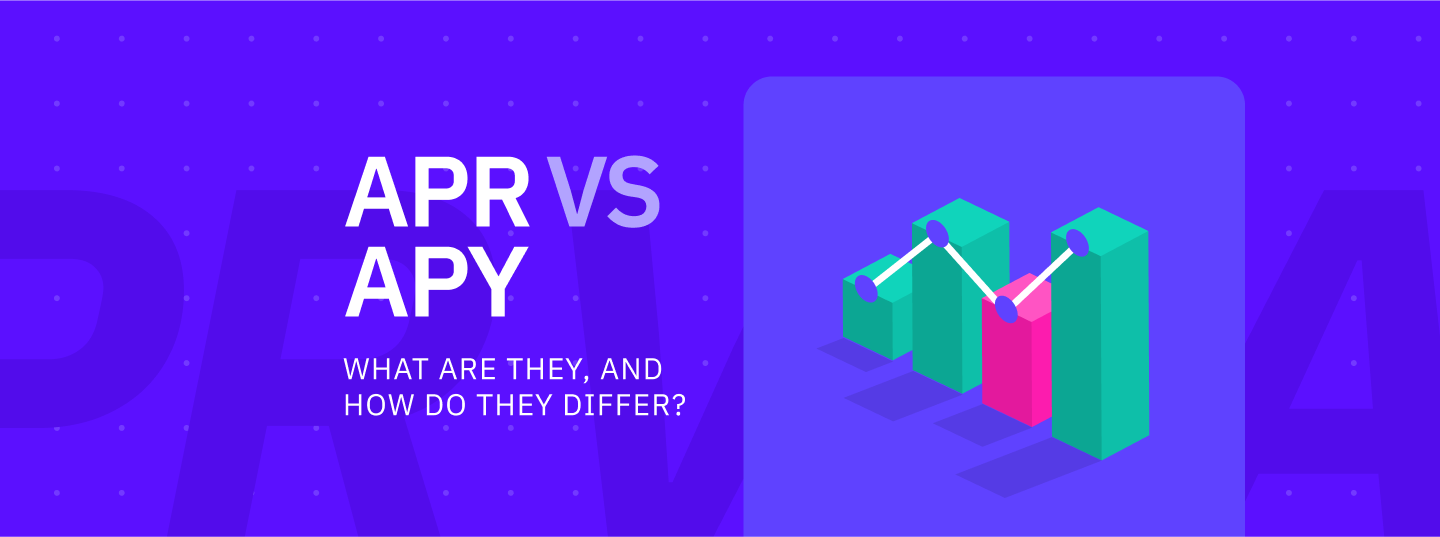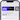# APR vs APY: What Are They, And How Do They Differ?

Nov 18,2022

There are a lot of acronyms and technical terms in the world of personal finance that can be confusing for new investors. Two of the most common terms you’ll see are APY and APR, and it’s important to understand the difference between them.

## Introduction: What is APR and APY?

The APR or annual percentage rate refers to the yearly interest generated that's charged to borrowers or paid to investors. Whenever you sign up for a loan or credit card with an APR (annual percentage rate), you are agreeing to pay the lender this particular interest rate over the course of one year.

As an example, if the APR is 3% and \$10,000 is borrowed, the initial \$10,000 loan plus \$300 in interest must be paid back after a year. In contrast, if \$10,000 is invested at the same interest rate, it will yield a \$300 return after a year.

It is clear from the above example that understanding the APR is important for determining how much money will be owed when borrowing money or how much an investor will receive. In the context of credit cards, APR is typically not charged when the card is used, but the balance must be paid in full by the due date. However, a balance that hasn't been paid by the due date is subject to interest (APR) at the end of each billing cycle.

Annual percentage yield, or APY, is a method of determining how much money can be earned on an interest-bearing account in one year. When compared to the APR, which only considers ordinary interest, the APY also takes compound interest into account. The compound interest earned is the sum of the interest earned and the principal invested. In this sense, the APY is more profitable than the APR if you invest your money.

## What is APR in crypto?

When it comes to cryptocurrency, the APR refers to the interest rate investors can expect to earn with their investment – whether it is through lending, staking, or liquidity mining. In addition, it takes into account other fees that borrowers have to pay, with the exception of compound interest.

An investment or loan's APR is essentially its ordinary interest rate applied to the principal. APRs are annualized rates, which means prorated interest will be charged if a loan or investment lasts for less than one year. For example, if you invest at 5% APR for six months, you will earn 2.5% of your original investment.

It is very straightforward to calculate the APR. Consider an investment of 0.5 Bitcoin (BTC) into Cake DeFi's Earn product. With an APR of 7%, after one year of investing, 0.035 Bitcoin will be earned on top of the initial investment. Consequently, the investment now totals 0.535 Bitcoin, which includes 0.5 Bitcoin principal and 0.035 Bitcoin interest (based on Earn's 7% APR).

## How to Calculate the Annual Percentage Rate?

In order to calculate the total final amount based on APR, follow these steps:

A = [P x (1 + RxT)] where

A = total amount
P = principal (initial investment)
R = interest rate
T = time in years

In the example above, it would be calculated as follows:

A = [P x (1 + RxT)] where

P = 0.5 BTC
R = 7% or 0.07
T = 1 year

A = [0.5 x (1 + 0.07x1)]
A = 0.535

If the investment is retained for a shorter period, the calculation changes. If you hold for three months, you will have held for a quarter of a year (0.25), so you will need to calculate:

A = [P x (1 + RxT)] where

P = 0.5 BTC
R = 7% or 0.07
T = 0.25 years

A = [0.5 x (1 + 0.07x0.25)]
A = 0.50875

That means that you will earn 0.00875 Bitcoin on top of the initial investment if you hold for three months.

## What is APY in crypto?

The Annual Percentage Yield, or APY, measures how much money can be earned on an interest-bearing account in a year. A crypto investment's APY indicates its rate of return. Unlike APR, which considers only the simple interest, APY takes compound interest into account.

Compund interest is the amount received both on the principal (the money you put into the account) and on the accumulated interest. The power of compounding lies in its ability to create money over time, which is why it is such a powerful investment tool. A compound interest rate is not the same as a simple interest rate. The term "simple interest" refers to interest that is generated only on the main deposit.

For crypto investors looking to make a return on investment while holding their investments, cryptocurrency savings or investment accounts with APY could be a good option. There are many crypto yield programs to choose from. Be sure to research them before signing up. There may be differences between platforms in terms of fees, entrance barriers, interest-earning procedures, and types of crypto assets available.

Cake DeFi, for instance, offers a varity of cryptocurrency investment options such as Earn, Lending, Staking or Liquidity Mining. Earn, for instance, can be compared to a savings account where you deposit Bitcoin, or any other coin, and get a fixed return after a certain period. Investing 10,000 USDT at 5% APY will earn you 10,500 USDT in a year, for instance. It is typical for interest to accrue regularly, whether it is daily, weekly, monthly, or on another schedule.

## How to Calculate the Annual Percentage Yield?

The APY calculation is a bit more complicated than the APR since interest is added to the principal, and then interest is calculated based on the number of periods the amount has been adjusted. The compound interest can be set on a daily, weekly, monthly, annual or perpetual basis.

APY = (1 + r / n)^n - 1 where

r = period rate
n = number of compounding periods

Suppose an investment of 1,000 DFI is made at a compound interest rate of 20% and daily compounding. As a result of the calculation above, an initial investment of 1,000 DFI yields 221 DFI over one year, making a total of 1,221 DFI in the first year and 1,492 the following year. Additionally,  higher interest rates and longer holding periods increase earnings.

## APR vs. APY: Which one is better?

As a general rule, APR rates are better when borrowing money, whereas APY rates are better when investing. If you are investing in crypto for yield, compounding can work wonders for you.

With Cake DeFi’s curated product line, you can invest a variety of popular coins with interest paid in APY in a low-risk, guaranteed-withdrawal environment. Some coins like DFI offer an APY rate of close to 18 percent. These investments offer some of the highest APYs in the industry and the platform itself ensures that your investment is always withdrawable.